x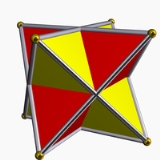TetrahedronOverview

In geometry
Geometry
Geometry arose as the field of knowledge dealing with spatial relationships. Geometry was one of the two fields of pre-modern mathematics, the other being the study of numbers ....

, a tetrahedron (plural: tetrahedra) is a polyhedron
Polyhedron
In elementary geometry a polyhedron is a geometric solid in three dimensions with flat faces and straight edges...

composed of four triangular
Triangle
A triangle is one of the basic shapes of geometry: a polygon with three corners or vertices and three sides or edges which are line segments. A triangle with vertices A, B, and C is denoted ....

faces, three of which meet at each vertex
Vertex (geometry)
In geometry, a vertex is a special kind of point that describes the corners or intersections of geometric shapes.-Of an angle:...

. A regular tetrahedron is one in which the four triangles are regular, or "equilateral", and is one of the Platonic solid
Platonic solid
In geometry, a Platonic solid is a convex polyhedron that is regular, in the sense of a regular polygon. Specifically, the faces of a Platonic solid are congruent regular polygons, with the same number of faces meeting at each vertex; thus, all its edges are congruent, as are its vertices and...

s. The tetrahedron is the only convex polyhedron
Convex polytope
A convex polytope is a special case of a polytope, having the additional property that it is also a convex set of points in the n-dimensional space Rn...

that has four faces.

The tetrahedron is the three-dimensional case of the more general concept of a Euclidean
Euclidean geometry
Euclidean geometry is a mathematical system attributed to the Alexandrian Greek mathematician Euclid, which he described in his textbook on geometry: the Elements. Euclid's method consists in assuming a small set of intuitively appealing axioms, and deducing many other propositions from these...

simplex
Simplex
In geometry, a simplex is a generalization of the notion of a triangle or tetrahedron to arbitrary dimension. Specifically, an n-simplex is an n-dimensional polytope which is the convex hull of its n + 1 vertices. For example, a 2-simplex is a triangle, a 3-simplex is a tetrahedron,...

.

The tetrahedron is one kind of pyramid
Pyramid (geometry)
In geometry, a pyramid is a polyhedron formed by connecting a polygonal base and a point, called the apex. Each base edge and apex form a triangle. It is a conic solid with polygonal base....

, which is a polyhedron with a flat polygon base and triangular faces connecting the base to a common point.DiscussionsHow many straight edges does a triangular pyramid have?Encyclopedia
In geometry
Geometry
Geometry arose as the field of knowledge dealing with spatial relationships. Geometry was one of the two fields of pre-modern mathematics, the other being the study of numbers ....

, a tetrahedron (plural: tetrahedra) is a polyhedron
Polyhedron
In elementary geometry a polyhedron is a geometric solid in three dimensions with flat faces and straight edges...

composed of four triangular
Triangle
A triangle is one of the basic shapes of geometry: a polygon with three corners or vertices and three sides or edges which are line segments. A triangle with vertices A, B, and C is denoted ....

faces, three of which meet at each vertex
Vertex (geometry)
In geometry, a vertex is a special kind of point that describes the corners or intersections of geometric shapes.-Of an angle:...

. A regular tetrahedron is one in which the four triangles are regular, or "equilateral", and is one of the Platonic solid
Platonic solid
In geometry, a Platonic solid is a convex polyhedron that is regular, in the sense of a regular polygon. Specifically, the faces of a Platonic solid are congruent regular polygons, with the same number of faces meeting at each vertex; thus, all its edges are congruent, as are its vertices and...

s. The tetrahedron is the only convex polyhedron
Convex polytope
A convex polytope is a special case of a polytope, having the additional property that it is also a convex set of points in the n-dimensional space Rn...

that has four faces.

The tetrahedron is the three-dimensional case of the more general concept of a Euclidean
Euclidean geometry
Euclidean geometry is a mathematical system attributed to the Alexandrian Greek mathematician Euclid, which he described in his textbook on geometry: the Elements. Euclid's method consists in assuming a small set of intuitively appealing axioms, and deducing many other propositions from these...

simplex
Simplex
In geometry, a simplex is a generalization of the notion of a triangle or tetrahedron to arbitrary dimension. Specifically, an n-simplex is an n-dimensional polytope which is the convex hull of its n + 1 vertices. For example, a 2-simplex is a triangle, a 3-simplex is a tetrahedron,...

.

The tetrahedron is one kind of pyramid
Pyramid (geometry)
In geometry, a pyramid is a polyhedron formed by connecting a polygonal base and a point, called the apex. Each base edge and apex form a triangle. It is a conic solid with polygonal base....

, which is a polyhedron with a flat polygon base and triangular faces connecting the base to a common point. In the case of a tetrahedron the base is a triangle (any of the four faces can be considered the base), so a tetrahedron is also known as a triangular pyramid.

Like all convex polyhedra, a tetrahedron can be folded from a single sheet of paper. It has two nets.

For any tetrahedron there exists a sphere (the circumsphere) such that the tetrahedron's vertices lie on the sphere's surface.

## Formulas for regular tetrahedron

For a regular tetrahedron of edge length:
 Base plane areaSurface area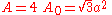HeightVolumeVolumeVolume is the quantity of three-dimensional space enclosed by some closed boundary, for example, the space that a substance or shape occupies or contains....Angle between an edge and a face(approx. 54.7356°) Angle between two faces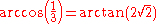(approx. 70.5288°) Angle between the segments joining the center and the vertices, also known as the tetrahedral angle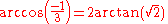(approx. 109.4712°) Solid angleSolid angleThe solid angle, Ω, is the two-dimensional angle in three-dimensional space that an object subtends at a point. It is a measure of how large that object appears to an observer looking from that point... at a vertex subtended by a face(approx. 0.55129 steradianSteradianThe steradian is the SI unit of solid angle. It is used to describe two-dimensional angular spans in three-dimensional space, analogous to the way in which the radian describes angles in a plane...s) Radius of circumsphere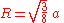Radius of insphere that is tangent to facesRadius of midsphereMidsphereIn geometry, the midsphere or intersphere of a polyhedron is a sphere which is tangent to every edge of the polyhedron. That is to say, it touches any given edge at exactly one point... that is tangent to edges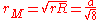Radius of exspheresDistance to exsphere center from a vertex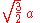Note that with respect to the base plane the slope
Slope
In mathematics, the slope or gradient of a line describes its steepness, incline, or grade. A higher slope value indicates a steeper incline....

of a face () is twice that of an edge (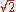), corresponding to the fact that the horizontal distance covered from the base to the apex
Apex (geometry)
In geometry, an apex is the vertex which is in some sense the highest of the figure to which it belongs.*In an isosceles triangle, the apex is the vertex where the two sides of equal length meet, opposite the unequal third side....

along an edge is twice that along the median
Median (geometry)
In geometry, a median of a triangle is a line segment joining a vertex to the midpoint of the opposing side. Every triangle has exactly three medians; one running from each vertex to the opposite side...

of a face. In other words, if C is the centroid
Centroid
In geometry, the centroid, geometric center, or barycenter of a plane figure or two-dimensional shape X is the intersection of all straight lines that divide X into two parts of equal moment about the line. Informally, it is the "average" of all points of X...

of the base, the distance from C to a vertex of the base is twice that from C to the midpoint of an edge of the base. This follows from the fact that the medians of a triangle intersect at its centroid, and this point divides each of them in two segments, one of which is twice as long as the other (see proof).

## Volume

The volume of a tetrahedron is given by the pyramid volume formula:where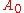is the area of the base and h the height from the base to the apex. This applies for each of the four choices of the base, so the distances from the apexes to the opposite faces are inversely proportional to the areas of these faces.

For a tetrahedron with vertices
a = (a1, a2, a3),
b = (b1, b2, b3),
c = (c1, c2, c3), and
d = (d1, d2, d3), the volume is (1/6)·|det
Determinant
In linear algebra, the determinant is a value associated with a square matrix. It can be computed from the entries of the matrix by a specific arithmetic expression, while other ways to determine its value exist as well...

(ab, bc, cd)|, or any other combination of pairs of vertices that form a simply connected graph
Graph theory
In mathematics and computer science, graph theory is the study of graphs, mathematical structures used to model pairwise relations between objects from a certain collection. A "graph" in this context refers to a collection of vertices or 'nodes' and a collection of edges that connect pairs of...

. This can be rewritten using a dot product
Dot product
In mathematics, the dot product or scalar product is an algebraic operation that takes two equal-length sequences of numbers and returns a single number obtained by multiplying corresponding entries and then summing those products...

and a cross product
Cross product
In mathematics, the cross product, vector product, or Gibbs vector product is a binary operation on two vectors in three-dimensional space. It results in a vector which is perpendicular to both of the vectors being multiplied and normal to the plane containing them...

, yieldingIf the origin of the coordinate system is chosen to coincide with vertex d, then d = 0, sowhere a, b, and c represent three edges that meet at one vertex, and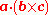is a scalar triple product. Comparing this formula with that used to compute the volume of a parallelepiped
Parallelepiped
In geometry, a parallelepiped is a three-dimensional figure formed by six parallelograms. By analogy, it relates to a parallelogram just as a cube relates to a square. In Euclidean geometry, its definition encompasses all four concepts...

, we conclude that the volume of a tetrahedron is equal to 1/6 of the volume of any parallelepiped that shares three converging edges with it.

The triple scalar can be represented by the following determinants: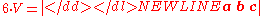or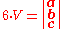where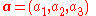is expressed as a row or column vector etc.
Hencewhere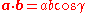etc.
which gives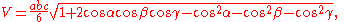where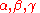are the plane angles occurring in vertex d. The angleis the angle between the two edges connecting the vertex d to the vertices b and c. The angledoes so for the vertices a and c, while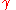is defined by the position of the vertices a and b.

Given the distances between the vertices of a tetrahedron the volume can be computed using the Cayley–Menger determinant: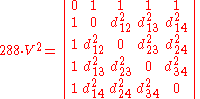where the subscripts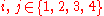represent the vertices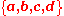andis the pairwise distance between them—i.e., the length of the edge connecting the two vertices. A negative value of the determinant means that a tetrahedron cannot be constructed with the given distances. This formula, sometimes called Tartaglia's formula, is essentially due to the painter Piero della Francesca
Piero della Francesca
Piero della Francesca was a painter of the Early Renaissance. As testified by Giorgio Vasari in his Lives of the Artists, to contemporaries he was also known as a mathematician and geometer. Nowadays Piero della Francesca is chiefly appreciated for his art. His painting was characterized by its...

in the 15th century, as a three dimensional analogue of the 1st century Heron's formula for the area of a triangle.

## Distance between the edges

Any two opposite edges of a tetrahedron lie on two skew lines
Skew lines
In solid geometry, skew lines are two lines that do not intersect and are not parallel. Equivalently, they are lines that are not coplanar. A simple example of a pair of skew lines is the pair of lines through opposite edges of a regular tetrahedron...

. If the closest pair of points between these two lines are points in the edges, they define the distance between the edges; otherwise, the distance between the edges equals that between one of the endpoints and the opposite edge. Let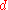be the distance between the skew lines formed by opposite edges a and b-c as calculated in. Then another volume formula is given by## Properties of a generalized tetrahedron

The tetrahedron has many properties analogous to those of a triangle, including an insphere, circumsphere, medial tetrahedron, and exspheres. It has respective centers such as incenter, circumcenter, excenters, Spieker center
Spieker circle
In geometry, the incircle of the medial triangle of a triangle ABC is the Spieker circle. Its center, the Spieker center, is the center of mass of the boundary of triangle ABC as well as being the incenter of the medial triangle....

and points such as a centroid. However, there is generally no orthocenter in the sense of intersecting altitudes. The circumsphere of the medial tetrahedron is analogous to the triangle's nine point circle, but does not generally pass through the base points of the altitudes of the reference tetrahedron.

Gaspard Monge
Gaspard Monge
Gaspard Monge, Comte de Péluse was a French mathematician, revolutionary, and was inventor of descriptive geometry. During the French Revolution, he was involved in the complete reorganization of the educational system, founding the École Polytechnique...

found a 'center' that exists in every tetrahedron, now known as the Monge point: the point where the six midplanes of a tetrahedron intersect. A midplane is defined as a plane that is orthogonal to an edge joining any two vertices that also contains the centroid of an opposite edge formed by joining the other two vertices. If the tetrahedron's altitudes do intersect, the Monge point and the orthocenter coincide.

An orthogonal line dropped from the Monge point to any face meets that face at the midpoint of the line segment between that face's orthocenter and the foot of the altitude dropped from the opposite vertex.

The centroid of a tetrahedron lies at the midpoint of its Monge point and circumcenter, on the Euler line of the tetrahedron.

The nine-point circle
Nine-point circle
In geometry, the nine-point circle is a circle that can be constructed for any given triangle. It is so named because it passes through nine significant points defined from the triangle...

of the general triangle has an analogue in the circumsphere of a tetrahedron's medial tetrahedron. It is the twelve-point sphere and besides the centroids of the four faces of the reference tetrahedron, it passes through four substitute Euler points, 1/3 of the way from the Monge point toward each of the four vertices. Finally it passes through the four base points of orthogonal lines dropped from each Euler point to the face not containing the vertex that generated the Euler point.

The center T of the twelve-point sphere also lies on the Euler line. Unlike its triangular counterpart, this center lies 1/3 of the way from the Monge point M towards the circumcenter. Also, an orthogonal line through T to a chosen face is coplanar with two other orthogonal lines to the same face. The first is an orthogonal line passing through the corresponding Euler point to the chosen face. The second is an orthogonal line passing through the centroid of the chosen face. This orthogonal line through the twelve-point center lies midway between the Euler point orthogonal line and the centroidal orthogonal line. Furthermore, for any face, the twelve-point center lies at the mid point of the corresponding Euler point and the orthocenter for that face.

The radius of the twelve-point sphere is 1/3 of the circumradius of the reference tetrahedron.

If OABC forms a generalized tetrahedron with a vertex O as the origin and vectors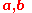andrepresent the positions of the vertices A, B, and C with respect to O, then the radius of the insphere is given by: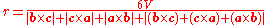and the radius of the circumsphere is given by: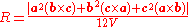which gives the radius of the twelve-point sphere:where: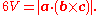In the formulas throughout this section, the scalar a² represents the inner vector product
Dot product
In mathematics, the dot product or scalar product is an algebraic operation that takes two equal-length sequences of numbers and returns a single number obtained by multiplying corresponding entries and then summing those products...

a·a; similarly b² and c².

The vector positions of various centers are as follows:

The centroid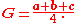The incenter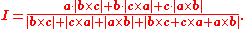The circumcenter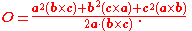The Monge pointThe Euler line relationships are: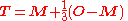where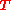is twelve-point center.

Also: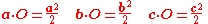and: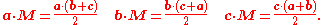## Geometric relations

A tetrahedron is a 3-simplex
Simplex
In geometry, a simplex is a generalization of the notion of a triangle or tetrahedron to arbitrary dimension. Specifically, an n-simplex is an n-dimensional polytope which is the convex hull of its n + 1 vertices. For example, a 2-simplex is a triangle, a 3-simplex is a tetrahedron,...

. Unlike the case of the other Platonic solids, all the vertices of a regular tetrahedron are equidistant from each other (they are the only possible arrangement of four equidistant points in 3-dimensional space).

A tetrahedron is a triangular pyramid
Pyramid (geometry)
In geometry, a pyramid is a polyhedron formed by connecting a polygonal base and a point, called the apex. Each base edge and apex form a triangle. It is a conic solid with polygonal base....

, and the regular tetrahedron is self-dual.

A regular tetrahedron can be embedded inside a cube in two ways such that each vertex is a vertex of the cube, and each edge is a diagonal of one of the cube's faces. For one such embedding, the Cartesian coordinates of the vertices
Vertex (geometry)
In geometry, a vertex is a special kind of point that describes the corners or intersections of geometric shapes.-Of an angle:...

are
(+1, +1, +1);
(−1, −1, +1);
(−1, +1, −1);
(+1, −1, −1).

This yields a tetrahedron with edge-length 2√2, centered at the origin. For the other tetrahedron (which is dual
Dual polyhedron
In geometry, polyhedra are associated into pairs called duals, where the vertices of one correspond to the faces of the other. The dual of the dual is the original polyhedron. The dual of a polyhedron with equivalent vertices is one with equivalent faces, and of one with equivalent edges is another...

to the first), reverse all the signs. These two tetrahedra's vertices combined are the vertices of a cube, demonstrating that the regular tetrahedron is the 3-demicube.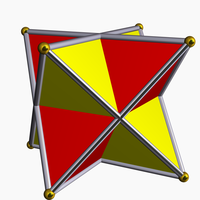The volume of this tetrahedron is 1/3 the volume of the cube. Combining both tetrahedra gives a regular polyhedral compound
Polyhedral compound
A polyhedral compound is a polyhedron that is itself composed of several other polyhedra sharing a common centre. They are the three-dimensional analogs of polygonal compounds such as the hexagram....

called the compound of two tetrahedra or stella octangula
Stella octangula
The stellated octahedron, or stella octangula, is the only stellation of the octahedron. It was named by Johannes Kepler in 1609, though it was known to earlier geometers...

.

The interior of the stella octangula is an octahedron
Octahedron
In geometry, an octahedron is a polyhedron with eight faces. A regular octahedron is a Platonic solid composed of eight equilateral triangles, four of which meet at each vertex....

, and correspondingly, a regular octahedron is the result of cutting off, from a regular tetrahedron, four regular tetrahedra of half the linear size (i.e., rectifying
Rectification (geometry)
In Euclidean geometry, rectification is the process of truncating a polytope by marking the midpoints of all its edges, and cutting off its vertices at those points...

the tetrahedron).

The above embedding divides the cube into five tetrahedra, one of which is regular. In fact, 5 is the minimum number of tetrahedra required to compose a cube.

Inscribing tetrahedra inside the regular compound of five cubes
Polyhedral compound
A polyhedral compound is a polyhedron that is itself composed of several other polyhedra sharing a common centre. They are the three-dimensional analogs of polygonal compounds such as the hexagram....

gives two more regular compounds, containing five and ten tetrahedra.

Regular tetrahedra cannot tessellate space
Honeycomb (geometry)
In geometry, a honeycomb is a space filling or close packing of polyhedral or higher-dimensional cells, so that there are no gaps. It is an example of the more general mathematical tiling or tessellation in any number of dimensions....

by themselves, although this result seems likely enough that Aristotle
Aristotle
Aristotle was a Greek philosopher and polymath, a student of Plato and teacher of Alexander the Great. His writings cover many subjects, including physics, metaphysics, poetry, theater, music, logic, rhetoric, linguistics, politics, government, ethics, biology, and zoology...

claimed it was possible. However, two regular tetrahedra can be combined with an octahedron, giving a rhombohedron
Rhombohedron
In geometry, a rhombohedron is a three-dimensional figure like a cube, except that its faces are not squares but rhombi. It is a special case of a parallelepiped where all edges are the same length....

that can tile space.

However, there is at least one irregular tetrahedron of which copies can tile space. If one relaxes the requirement that the tetrahedra be all the same shape, one can tile space using only tetrahedra in various ways. For example, one can divide an octahedron into four identical tetrahedra and combine them again with two regular ones. (As a side-note: these two kinds of tetrahedron have the same volume.)

The tetrahedron is unique among the uniform polyhedra
Uniform polyhedron
A uniform polyhedron is a polyhedron which has regular polygons as faces and is vertex-transitive...

in possessing no parallel faces.

### Related polyhedra

A truncation process applied to the tetrahedron produces a series of uniform polyhedra
Uniform polyhedron
A uniform polyhedron is a polyhedron which has regular polygons as faces and is vertex-transitive...

. Truncating edges down to points produces the octahedron
Octahedron
In geometry, an octahedron is a polyhedron with eight faces. A regular octahedron is a Platonic solid composed of eight equilateral triangles, four of which meet at each vertex....

as a rectified tetahedron. The process completes as a birectification, reducing the original faces down to points, and producing the self-dual tetrahedron once again.
This polyhedron is topologically related as a part of sequence of regular polyhedra with Schläfli symbols {3,n}, continuing into the hyperbolic plane
Hyperbolic space
In mathematics, hyperbolic space is a type of non-Euclidean geometry. Whereas spherical geometry has a constant positive curvature, hyperbolic geometry has a negative curvature: every point in hyperbolic space is a saddle point...

.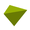{3,3}TetrahedronIn geometry, a tetrahedron is a polyhedron composed of four triangular faces, three of which meet at each vertex. A regular tetrahedron is one in which the four triangles are regular, or "equilateral", and is one of the Platonic solids...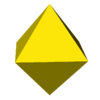{3,4}OctahedronIn geometry, an octahedron is a polyhedron with eight faces. A regular octahedron is a Platonic solid composed of eight equilateral triangles, four of which meet at each vertex....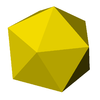{3,5}IcosahedronIn geometry, an icosahedron is a regular polyhedron with 20 identical equilateral triangular faces, 30 edges and 12 vertices. It is one of the five Platonic solids....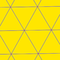{3,6}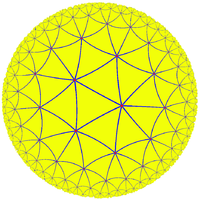{3,7}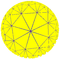{3,8}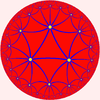{3,9}

### Intersecting tetrahedra

An interesting polyhedron can be constructed from five intersecting tetrahedra
Compound of five tetrahedra
This compound polyhedron is also a stellation of the regular icosahedron. It was first described by Edmund Hess in 1876.-As a compound:It can be constructed by arranging five tetrahedra in rotational icosahedral symmetry , as colored in the upper right model...

. This compound
Polyhedral compound
A polyhedral compound is a polyhedron that is itself composed of several other polyhedra sharing a common centre. They are the three-dimensional analogs of polygonal compounds such as the hexagram....

of five tetrahedra has been known for hundreds of years. It comes up regularly in the world of origami
Origami
is the traditional Japanese art of paper folding, which started in the 17th century AD at the latest and was popularized outside Japan in the mid-1900s. It has since then evolved into a modern art form...

. Joining the twenty vertices would form a regular dodecahedron. There are both left-handed
Left-handed
Left-handedness is the preference for the left hand over the right for everyday activities such as writing. In ancient times it was seen as a sign of the devil, and was abhorred in many cultures...

and right-handed forms, which are mirror image
Mirror image
A mirror image is a reflected duplication of an object that appears identical but reversed. As an optical effect it results from reflection off of substances such as a mirror or water. It is also a concept in geometry and can be used as a conceptualization process for 3-D structures...

s of each other.

### Isometries of regular tetrahedra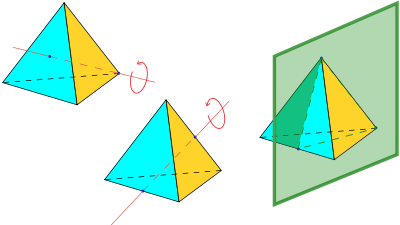The vertices of a cube
Cube
In geometry, a cube is a three-dimensional solid object bounded by six square faces, facets or sides, with three meeting at each vertex. The cube can also be called a regular hexahedron and is one of the five Platonic solids. It is a special kind of square prism, of rectangular parallelepiped and...

can be grouped into two groups of four, each forming a regular tetrahedron (see above, and also animation, showing one of the two tetrahedra in the cube). The symmetries
Symmetry in mathematics
Symmetry occurs not only in geometry, but also in other branches of mathematics. It is actually the same as invariance: the property that something does not change under a set of transformations....

of a regular tetrahedron correspond to half of those of a cube: those that map the tetrahedrons to themselves, and not to each other.

The tetrahedron is the only Platonic solid that is not mapped to itself by point inversion.

The regular tetrahedron has 24 isometries, forming the symmetry group
Symmetry group
The symmetry group of an object is the group of all isometries under which it is invariant with composition as the operation...

Td, isomorphic to S4
Symmetric group
In mathematics, the symmetric group Sn on a finite set of n symbols is the group whose elements are all the permutations of the n symbols, and whose group operation is the composition of such permutations, which are treated as bijective functions from the set of symbols to itself...

. They can be categorized as follows:
• T, isomorphic to alternating group A4 (the identity and 11 proper rotations) with the following conjugacy class
Conjugacy class
In mathematics, especially group theory, the elements of any group may be partitioned into conjugacy classes; members of the same conjugacy class share many properties, and study of conjugacy classes of non-abelian groups reveals many important features of their structure...

es (in parentheses are given the permutations of the vertices, or correspondingly, the faces, and the unit quaternion representation
Quaternions and spatial rotation
Unit quaternions provide a convenient mathematical notation for representing orientations and rotations of objects in three dimensions. Compared to Euler angles they are simpler to compose and avoid the problem of gimbal lock. Compared to rotation matrices they are more numerically stable and may...

):
• identity (identity; 1)
• rotation about an axis through a vertex, perpendicular to the opposite plane, by an angle of ±120°: 4 axes, 2 per axis, together 8 ((1 2 3), etc.; (1 ± i ± j ± k)/2)
• rotation by an angle of 180° such that an edge maps to the opposite edge: 3 ((1 2)(3 4), etc.; ijk)
• reflections in a plane perpendicular to an edge: 6
• reflections in a plane combined with 90° rotation about an axis perpendicular to the plane: 3 axes, 2 per axis, together 6; equivalently, they are 90° rotations combined with inversion (x is mapped to −x): the rotations correspond to those of the cube about face-to-face axes

### Isometries of irregular tetrahedra

The isometries of an irregular tetrahedron depend on the geometry of the tetrahedron, with 7 cases possible. In each case a 3-dimensional point group
Point groups in three dimensions
In geometry, a point group in three dimensions is an isometry group in three dimensions that leaves the origin fixed, or correspondingly, an isometry group of a sphere. It is a subgroup of the orthogonal group O, the group of all isometries that leave the origin fixed, or correspondingly, the group...

is formed.
• An equilateral triangle base and isosceles (and non-equilateral) triangle sides gives 6 isometries, corresponding to the 6 isometries of the base. As permutations of the vertices, these 6 isometries are the identity 1, (123), (132), (12), (13) and (23), forming the symmetry group C3v, isomorphic to S3.
• Four congruent isosceles (non-equilateral) triangles gives 8 isometries. If edges (1,2) and (3,4) are of different length to the other 4 then the 8 isometries are the identity 1, reflections (12) and (34), and 180° rotations (12)(34), (13)(24), (14)(23) and improper 90° rotations (1234) and (1432) forming the symmetry group D2d.
• Four congruent scalene triangles gives 4 isometries. The isometries are 1 and the 180° rotations (12)(34), (13)(24), (14)(23). This is the Klein four-group
Klein four-group
In mathematics, the Klein four-group is the group Z2 × Z2, the direct product of two copies of the cyclic group of order 2...

V4Z22, present as the point group D2. A tetrahedron with this symmetry is called disphenoid
Disphenoid
A disphenoid is a polyhedron whose four faces are identical triangles. The faces of a tetragonal disphenoid are isosceles; the faces of a rhombic disphenoid are scalene. If the faces are equilateral triangles, one obtains a regular tetrahedron, which is not normally considered a disphenoid.All the...

.
• Two pairs of isomorphic isosceles (non-equilateral) triangles. This gives two opposite edges (1,2) and (3,4) that are perpendicular but different lengths, and then the 4 isometries are 1, reflections (12) and (34) and the 180° rotation (12)(34). The symmetry group is C2v, isomorphic to V4.
• Two pairs of isomorphic scalene triangles. This has two pairs of equal edges (1,3), (2,4) and (1,4), (2,3) but otherwise no edges equal. The only two isometries are 1 and the rotation (12)(34), giving the group C2 isomorphic to Z2.
• Two unequal isosceles triangles with a common base. This has two pairs of equal edges (1,3), (1,4) and (2,3), (2,4) and otherwise no edges equal. The only two isometries are 1 and the reflection (34), giving the group Cs isomorphic to Z2.
• No edges equal, so that the only isometry is the identity, and the symmetry group is the trivial group.

## A law of sines for tetrahedra and the space of all shapes of tetrahedra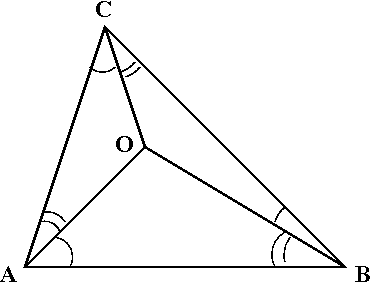A corollary of the usual law of sines
Law of sines
In trigonometry, the law of sines is an equation relating the lengths of the sides of an arbitrary triangle to the sines of its angles...

is that in a tetrahedron with vertices O, A, B, C, we haveOne may view the two sides of this identity as corresponding to clockwise and counterclockwise orientations of the surface.

Putting any of the four vertices in the role of O yields four such identities, but in a sense at most three of them are independent: If the "clockwise" sides of three of them are multiplied and the product is inferred to be equal to the product of the "counterclockwise" sides of the same three identities, and then common factors are cancelled from both sides, the result is the fourth identity. One reason to be interested in this "independence" relation is this: It is widely known that three angles are the angles of some triangle if and only if their sum is 180° (π radians). What condition on 12 angles is necessary and sufficient for them to be the 12 angles of some tetrahedron? Clearly the sum of the angles of any side of the tetrahedron must be 180°. Since there are four such triangles, there are four such constraints on sums of angles, and the number of degrees of freedom
Degrees of freedom (statistics)
In statistics, the number of degrees of freedom is the number of values in the final calculation of a statistic that are free to vary.Estimates of statistical parameters can be based upon different amounts of information or data. The number of independent pieces of information that go into the...

is thereby reduced from 12 to 8. The four relations given by this sine law further reduce the number of degrees of freedom, not from 8 down to 4, but only from 8 down to 5, since the fourth constraint is not independent of the first three. Thus the space of all shapes of tetrahedra is 5-dimensional.

## Applications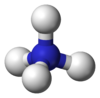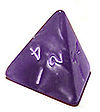### Numerical analysis

In numerical analysis
Numerical analysis
Numerical analysis is the study of algorithms that use numerical approximation for the problems of mathematical analysis ....

, complicated three-dimensional shapes are commonly broken down into, or approximated
Approximation
An approximation is a representation of something that is not exact, but still close enough to be useful. Although approximation is most often applied to numbers, it is also frequently applied to such things as mathematical functions, shapes, and physical laws.Approximations may be used because...

by, a polygonal mesh
Polygon mesh
A polygon mesh or unstructured grid is a collection of vertices, edges and faces that defines the shape of a polyhedral object in 3D computer graphics and solid modeling...

of irregular tetrahedra in the process of setting up the equations for finite element analysis especially in the numerical solution of partial differential equations. These methods have wide applications in practical applications in computational fluid dynamics
Computational fluid dynamics
Computational fluid dynamics, usually abbreviated as CFD, is a branch of fluid mechanics that uses numerical methods and algorithms to solve and analyze problems that involve fluid flows. Computers are used to perform the calculations required to simulate the interaction of liquids and gases with...

, aerodynamics
Aerodynamics
Aerodynamics is a branch of dynamics concerned with studying the motion of air, particularly when it interacts with a moving object. Aerodynamics is a subfield of fluid dynamics and gas dynamics, with much theory shared between them. Aerodynamics is often used synonymously with gas dynamics, with...

, electromagnetic field
Electromagnetic field
An electromagnetic field is a physical field produced by moving electrically charged objects. It affects the behavior of charged objects in the vicinity of the field. The electromagnetic field extends indefinitely throughout space and describes the electromagnetic interaction...

s, civil engineering
Civil engineering
Civil engineering is a professional engineering discipline that deals with the design, construction, and maintenance of the physical and naturally built environment, including works like roads, bridges, canals, dams, and buildings...

, chemical engineering
Chemical engineering
Chemical engineering is the branch of engineering that deals with physical science , and life sciences with mathematics and economics, to the process of converting raw materials or chemicals into more useful or valuable forms...

, naval architecture and engineering
Naval architecture
Naval architecture is an engineering discipline dealing with the design, construction, maintenance and operation of marine vessels and structures. Naval architecture involves basic and applied research, design, development, design evaluation and calculations during all stages of the life of a...

, and related fields.

### ChemistryChemistryChemistry is the science of matter, especially its chemical reactions, but also its composition, structure and properties. Chemistry is concerned with atoms and their interactions with other atoms, and particularly with the properties of chemical bonds....

The tetrahedron shape is seen in nature in covalent bonds of molecules. All sp3-hybridized atoms are surrounded by atoms lying in each corner of a tetrahedron. For instance in a methane
Methane
Methane is a chemical compound with the chemical formula . It is the simplest alkane, the principal component of natural gas, and probably the most abundant organic compound on earth. The relative abundance of methane makes it an attractive fuel...

molecule (CH4) or an ammonium
Ammonium
The ammonium cation is a positively charged polyatomic cation with the chemical formula NH. It is formed by the protonation of ammonia...

ion (NH4+), four hydrogen atoms surround a central carbon or nitrogen atom with tetrahedral symmetry. For this reason, one of the leading journals in organic chemistry is called Tetrahedron. See also tetrahedral molecular geometry
Tetrahedral molecular geometry
In a tetrahedral molecular geometry a central atom is located at the center with four substituents that are located at the corners of a tetrahedron. The bond angles are cos−1 ≈ 109.5° when all four substituents are the same, as in CH4. This molecular geometry is common throughout the first...

. The central angle
Central angle
A central angle is an angle which vertex is the center of a circle, and whose sides pass through a pair of points on the circle, thereby subtending an arc between those two points whose angle is equal to the central angle itself...

between any two vertices of a perfect tetrahedron is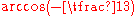, or approximately 109.47°).

Water
Water
Water is a chemical substance with the chemical formula H2O. A water molecule contains one oxygen and two hydrogen atoms connected by covalent bonds. Water is a liquid at ambient conditions, but it often co-exists on Earth with its solid state, ice, and gaseous state . Water also exists in a...

, H2O, also has a tetrahedral structure, with two hydrogen atoms and two lone pairs of electrons around the central oxygen atoms. Its tetrahedral symmetry is not perfect, however, because the lone pairs repel more than the single O-H bonds.

Quaternary phase diagrams in chemistry are represented graphically as tetrahedra.

However, quaternary phase diagrams in communication engineering are represented graphically on a two-dimensional plane.

### ElectricityElectricityElectricity is a general term encompassing a variety of phenomena resulting from the presence and flow of electric charge. These include many easily recognizable phenomena, such as lightning, static electricity, and the flow of electrical current in an electrical wire... and electronicsElectronicsElectronics is the branch of science, engineering and technology that deals with electrical circuits involving active electrical components such as vacuum tubes, transistors, diodes and integrated circuits, and associated passive interconnection technologies...

If six equal resistor
Resistor
A linear resistor is a linear, passive two-terminal electrical component that implements electrical resistance as a circuit element.The current through a resistor is in direct proportion to the voltage across the resistor's terminals. Thus, the ratio of the voltage applied across a resistor's...

s are solder
Solder
Solder is a fusible metal alloy used to join together metal workpieces and having a melting point below that of the workpiece.Soft solder is what is most often thought of when solder or soldering are mentioned and it typically has a melting range of . It is commonly used in electronics and...

ed together to form a tetrahedron, then the resistance measured between any two vertices is half that of one resistor.

Since silicon
Silicon
Silicon is a chemical element with the symbol Si and atomic number 14. A tetravalent metalloid, it is less reactive than its chemical analog carbon, the nonmetal directly above it in the periodic table, but more reactive than germanium, the metalloid directly below it in the table...

is the most common semiconductor
Semiconductor
A semiconductor is a material with electrical conductivity due to electron flow intermediate in magnitude between that of a conductor and an insulator. This means a conductivity roughly in the range of 103 to 10−8 siemens per centimeter...

used in solid-state electronics, and silicon has a valence
Valence (chemistry)
In chemistry, valence, also known as valency or valence number, is a measure of the number of bonds formed by an atom of a given element. "Valence" can be defined as the number of valence bonds...

of four, the tetrahedral shape of the four chemical bonds in silicon is a strong influence on how crystal
Crystal
A crystal or crystalline solid is a solid material whose constituent atoms, molecules, or ions are arranged in an orderly repeating pattern extending in all three spatial dimensions. The scientific study of crystals and crystal formation is known as crystallography...

s of silicon form and what shapes they assume.

### GameGameA game is structured playing, usually undertaken for enjoyment and sometimes used as an educational tool. Games are distinct from work, which is usually carried out for remuneration, and from art, which is more often an expression of aesthetic or ideological elements...s

Especially in roleplaying, this solid is known as a 4-sided die
4-sided die
Four-sided dice are often used in role-playing games, such as Dungeons & Dragons, to get small numbers for things such as damage or character statistic increases. Each die is a tetrahedron with 4 equilateral triangle-shaped faces. This type of die does not roll well and is thus usually thrown into...

, one of the more common polyhedral dice, with the number rolled appearing around the bottom or on the top vertex.
Some Rubik's Cube
Rubik's Cube
Rubik's Cube is a 3-D mechanical puzzle invented in 1974 by Hungarian sculptor and professor of architecture Ernő Rubik.Originally called the "Magic Cube", the puzzle was licensed by Rubik to be sold by Ideal Toy Corp. in 1980 and won the German Game of the Year special award for Best Puzzle that...

-like puzzles are tetrahedral, such as the Pyraminx
Pyraminx
The Pyraminx is a tetrahedral puzzle similar to the Rubik's Cube. It was invented and patented by Uwe Meffert, and introduced by Tomy Toys of Japan in 1981. Meffert continues to sell it in his toy shop, ....

and Pyramorphix
Pyramorphix
The Pyramorphix is a tetrahedral puzzle similar to the Rubik's Cube. It has a total of 8 movable pieces to rearrange, compared to the 20 of the Rubik's cube. Though it looks like a simpler version of the Pyraminx, it is an edge-turning puzzle with the mechanism identical to that of the Pocket...

.

### Color spaceColor spaceA color model is an abstract mathematical model describing the way colors can be represented as tuples of numbers, typically as three or four values or color components...

Tetrahedra are used in color space conversion algorithms specifically for cases in which the luminance axis diagonally segments the color space (e.g. RGB, CMY).

### Contemporary ArtContemporary artContemporary art can be defined variously as art produced at this present point in time or art produced since World War II. The definition of the word contemporary would support the first view, but museums of contemporary art commonly define their collections as consisting of art produced...

The Austrian artist Martina Schettina
Martina Schettina
Martina Schettina is an Austrian artist.- Life :Martina Schettina was born in 1961 in the district Währing in Vienna. Her father was a Mathematician...

created a tetrahedron using fluorescent lamp
Fluorescent lamp
A fluorescent lamp or fluorescent tube is a gas-discharge lamp that uses electricity to excite mercury vapor. The excited mercury atoms produce short-wave ultraviolet light that then causes a phosphor to fluoresce, producing visible light. A fluorescent lamp converts electrical power into useful...

s. It was shown at the light art biennale Austria 2010.

It is used as album artwork, surrounded by black flames on The End of All Things to Come
The End of All Things to Come
The End of All Things to Come is the second studio album by American heavy metal band Mudvayne, released on November 19, 2002.It was certified Gold by the RIAA.- Musical style :...

by Mudvayne
Mudvayne
Mudvayne is an American heavy metal band. Their work is marked by the use of sonic experimentation, innovative album art, and elaborate visual appearance, which has included face and body paint, masks and uniforms...

.

### GeologyGeologyGeology is the science comprising the study of solid Earth, the rocks of which it is composed, and the processes by which it evolves. Geology gives insight into the history of the Earth, as it provides the primary evidence for plate tectonics, the evolutionary history of life, and past climates...

The tetrahedral hypothesis
Tetrahedral hypothesis
The Tetrahedral hypothesis is an obsolete scientific theory attempting to explain the arrangement of the Earth's continents and oceans by referring to the geometry of a tetrahedron...

William Lowthian Green
William Lowthian Green was an English adventurer and merchant, who later became cabinet minister in the Kingdom of Hawaii. As an amateur geologist, he published a theory of the formation of the earth called the tetrahedral hypothesis.-Life:...

to explain the formation of the Earth, was popular through the early 20th century.

• Boerdijk–Coxeter helix
Boerdijk–Coxeter helix
The Boerdijk–Coxeter helix, named after H. S. M. Coxeter and A. H. Boerdijk, is a linear stacking of regular tetrahedra. There are two chiral forms, with either clockwise or counterclockwise windings. Contrary to any other stacking of Platonic solids, the Boerdijk–Coxeter helix is not rotationally...

• Caltrop
Caltrop
A caltrop is an antipersonnel weapon made up of two or more sharp nails or spines arranged in such a manner that one of them always points upward from a stable base...

• Demihypercube
• Disphenoid
Disphenoid
A disphenoid is a polyhedron whose four faces are identical triangles. The faces of a tetragonal disphenoid are isosceles; the faces of a rhombic disphenoid are scalene. If the faces are equilateral triangles, one obtains a regular tetrahedron, which is not normally considered a disphenoid.All the...

— a tetrahedron with mirror symmetry
• Hill tetrahedron
Hill tetrahedron
In geometry, the Hill tetrahedra are a family of space-filling tetrahedra. They were discovered in 1896 by M.J.M. Hill, a professor of mathematics at the University College London, who showed that they are scissor-congruent to a cube.- Construction :...

• Simplex
Simplex
In geometry, a simplex is a generalization of the notion of a triangle or tetrahedron to arbitrary dimension. Specifically, an n-simplex is an n-dimensional polytope which is the convex hull of its n + 1 vertices. For example, a 2-simplex is a triangle, a 3-simplex is a tetrahedron,...

• Tetra-Pak
• Tetrahedral kite
Tetrahedral kite
A tetrahedral kite is a multicelled rigid box kite composed of tetrahedrally shaped cells. The cells are usually arranged in such a way that the entire kite is also a regular tetrahedron. The kite can be described as a compound dihedral kite as well....

• Tetrahedral number
Tetrahedral number
A tetrahedral number, or triangular pyramidal number, is a figurate number that represents a pyramid with a triangular base and three sides, called a tetrahedron...

• Triangular dipyramid
Triangular dipyramid
In geometry, the triangular bipyramid is the first in the infinite set of face-transitive bipyramids. It is the dual of the triangular prism with 6 isosceles triangle faces....

— constructed by joining two tetrahedra along one face
• Tetrahedron packing
Tetrahedron packing
In geometry, tetrahedron packing is the problem of arranging identical regular tetrahedra throughout three-dimensional space so as to fill the maximum possible fraction of space....